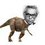# Mehul's doubt.

If $a=8-4\sqrt{2}$ then find the value of :

$\dfrac{a+2}{a-2} - \dfrac{a+2\sqrt{2}}{a-2\sqrt{2}}$Note by Nihar Mahajan
6 years, 2 months ago

This discussion board is a place to discuss our Daily Challenges and the math and science related to those challenges. Explanations are more than just a solution — they should explain the steps and thinking strategies that you used to obtain the solution. Comments should further the discussion of math and science.

When posting on Brilliant:

• Use the emojis to react to an explanation, whether you're congratulating a job well done , or just really confused .
• Ask specific questions about the challenge or the steps in somebody's explanation. Well-posed questions can add a lot to the discussion, but posting "I don't understand!" doesn't help anyone.
• Try to contribute something new to the discussion, whether it is an extension, generalization or other idea related to the challenge.

MarkdownAppears as
*italics* or _italics_ italics
**bold** or __bold__ bold
- bulleted- list
• bulleted
• list
1. numbered2. list
1. numbered
2. list
Note: you must add a full line of space before and after lists for them to show up correctly
paragraph 1paragraph 2

paragraph 1

paragraph 2

[example link](https://brilliant.org)example link
> This is a quote
This is a quote
    # I indented these lines
# 4 spaces, and now they show
# up as a code block.

print "hello world"
# I indented these lines
# 4 spaces, and now they show
# up as a code block.

print "hello world"
MathAppears as
Remember to wrap math in $$ ... $$ or $ ... $ to ensure proper formatting.
2 \times 3 $2 \times 3$
2^{34} $2^{34}$
a_{i-1} $a_{i-1}$
\frac{2}{3} $\frac{2}{3}$
\sqrt{2} $\sqrt{2}$
\sum_{i=1}^3 $\sum_{i=1}^3$
\sin \theta $\sin \theta$
\boxed{123} $\boxed{123}$

Sort by:

\begin{aligned} \dfrac{a+2}{a-2} - \dfrac{a+2\sqrt{2}}{a-2\sqrt{2}} & = \dfrac{8-4\sqrt{2}+2}{8-4\sqrt{2}-2} - \dfrac{8-4\sqrt{2}+2\sqrt{2}}{8-4\sqrt{2}-2\sqrt{2}} \\ & = \dfrac{10-4\sqrt{2}}{6-4\sqrt{2}} - \dfrac{8-2\sqrt{2}}{8-6\sqrt{2}} \\ & = \dfrac{5-2\sqrt{2}}{3-2\sqrt{2}} - \dfrac{4-\sqrt{2}}{4-3\sqrt{2}} \\ & = \dfrac{(5-2\sqrt{2})(3+2\sqrt{2})}{9-8} - \dfrac{(4-\sqrt{2})(4+3\sqrt{2})}{16-18} \\ & = 15+4\sqrt{2} - 8 + \dfrac{16 + 8\sqrt{2} - 6}{2} \\ & = 7 +4\sqrt{2} + 5 + 4\sqrt{2} \\ & = 12+8\sqrt{2} \end{aligned}

- 6 years, 2 months ago

Err, sir, There is a typo in the line of the answer. It should be "+"

- 6 years, 2 months ago

Thanks.

- 6 years, 2 months ago

$\huge\ddot\smile$

- 6 years, 2 months ago

12+10\sqrt{2}

- 6 years, 2 months ago

@Soumya Khurana , I am afraid that there is no such option in the paper I got this from.

- 6 years, 2 months ago

Can u tell me the options @Mehul Arora

- 6 years, 2 months ago

Sure. a. $12+ \sqrt {2}$

b. $12- \sqrt {2}$

c. 2

d. -2

- 6 years, 2 months ago

@Mehul Arora , I am afraid that there is no such option in the paper which is the correct value of the expression above.

- 6 years, 2 months ago

There should be one more option as "None of the given". :P

- 6 years, 2 months ago

Yeah... I calculated it manually. :P

- 6 years, 2 months ago

Thanks! I just got juggled up with the signs.

$cheers!$

- 6 years, 2 months ago

@Mehul Arora I checked for sure using a claculator...None of the options match. :(

- 6 years, 2 months ago

According to the question, we have to find,

$\huge \dfrac{8-4\sqrt{2}+2}{8-4\sqrt{2}-2}-\dfrac{8-4\sqrt{2}+2\sqrt{2}}{8-4\sqrt{2}-2\sqrt{2}} \\ \huge =\dfrac{10-4\sqrt{2}}{6-4\sqrt{2}} \times \dfrac{6+4\sqrt{2}}{6+\sqrt{2}} - \dfrac{8-2\sqrt{2}}{8-6\sqrt{2}} \times \dfrac{8+6\sqrt{2}}{8+6\sqrt{2}} \\ \huge = 7+4\sqrt{2}-(-5-4\sqrt{2}) \\ \huge = \boxed{12+8\sqrt{2}}$

- 6 years, 2 months ago

You have made a mistake.Find it.

- 6 years, 2 months ago

Simply put the value of $a$ and calculate. :P

- 6 years, 2 months ago

The answer I am getting is $12 + 8\sqrt{2}$ , Is it correct?

- 6 years, 2 months ago

The correct ans is $12+8\sqrt{2}$.

- 6 years, 2 months ago

Yes , I am correct then. :P

- 6 years, 2 months ago

Yeah. Thank you sir. :)

I needed the answer. I found out the procedure.

Thanks everyone @Nihar Mahajan @Sravanth Chebrolu @Soumya Khurana and of course @Sandeep Bhardwaj sir.

- 6 years, 2 months ago

No need to @Mention!!! $\huge \ddot \smile$

- 6 years, 2 months ago

ha ha componendo dividendo solved it for me isnt that way easier???

- 5 years, 7 months ago

How did you solve this using componendo dividendo ? Also , post your solution.

- 5 years, 7 months ago

sorry i cant dont know a thing about latex.

- 5 years, 7 months ago

You can post a solution without latex too. I will understand it. Or at least post your approach.I am curious about this method.

- 5 years, 7 months ago